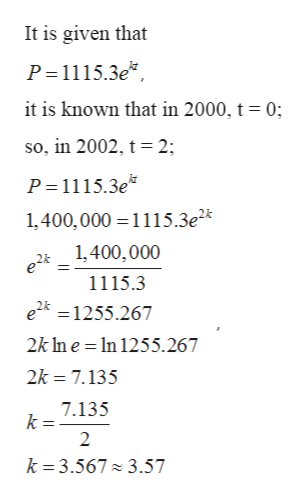# The populations P ( in thousands) of a certain city from 2000 through 2008 can be modeled by P = 1115.3ekt, where t is the year, with t=o corresponding to 2000. in 2002, the population was about 1,400,000. find the value of k for the model. Round Your result to four demical places

Question
48 views

The populations P ( in thousands) of a certain city from 2000 through 2008 can be modeled by P = 1115.3ekt, where t is the year, with t=o corresponding to 2000. in 2002, the population was about 1,400,000. find the value of k for the model. Round Your result to four demical places

check_circle

Step 1

Given: -

Step 2

To find: -

Step 3

Calculation...help_outlineImage TranscriptioncloseIt is given that P=1115.3e*, it is known that in 2000, t= 0; so, in 2002, t = 2; P=1115.3e* 1,400,000 =1115.3e2* 1,400,000 1115.3 e2k =1255.267 2k In e = In 1255.267 2k = 7.135 7.135 k : k = 3.567 3.57 fullscreen

### Want to see the full answer?

See Solution

#### Want to see this answer and more?

Solutions are written by subject experts who are available 24/7. Questions are typically answered within 1 hour.*

See Solution
*Response times may vary by subject and question.
Tagged in

### Other